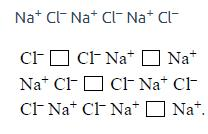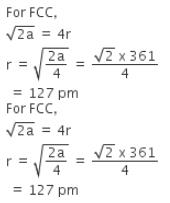Courses

# Test Level 1: Previous Year Questions- Solid State

## 7 Questions MCQ Test Chemistry Class 12 | Test Level 1: Previous Year Questions- Solid State

Description
This mock test of Test Level 1: Previous Year Questions- Solid State for Class 12 helps you for every Class 12 entrance exam. This contains 7 Multiple Choice Questions for Class 12 Test Level 1: Previous Year Questions- Solid State (mcq) to study with solutions a complete question bank. The solved questions answers in this Test Level 1: Previous Year Questions- Solid State quiz give you a good mix of easy questions and tough questions. Class 12 students definitely take this Test Level 1: Previous Year Questions- Solid State exercise for a better result in the exam. You can find other Test Level 1: Previous Year Questions- Solid State extra questions, long questions & short questions for Class 12 on EduRev as well by searching above.
QUESTION: 1

### The no. of atoms per unit cell in B.C.C. & F.C.C. is respectively -  [AIEEE-2002]

Solution:

B.C.C - Points are at corners and one in the centre of the unit cell.
Number of atoms per unit cell = 8 x 18 + 1 = 2.

F.C.C - Points are at the corners and also centre of the six faces of each cell.
Number of atoms per unit cell = 8 x 18 + 6 x 12 = 4.

QUESTION: 2

### How many unit cells are present in a cube-shaped ideal crystal of NaCl of mass 1.00 g? [Atomic masses: Na = 23, Cl = 35.5]  [AIEEE-2003]

Solution:

Mass (m) = density x volume = 1 g

Mol wt of NaCl = 58.5 g

Number of unit cell present in a cube shaped crystal of NaCl of mass 1 g = m x Na / (M x Z)

In NaCl each unit cell has 4 NaCl units.

Z = 4

QUESTION: 3

### What type of crystal defect is indicated in the diagram below ? [AIEEE-2004]Solution:

Here the crystal defect is the paired vacancies caused by the ions missing from their lattice sites.

QUESTION: 4

An ionic compound has a unit cell consisting of A ions at the corners of a cube and B ions on the centres of the faces of the cube. The empirical formula for this compound would be

[AIEEE-2005]

Solution:

Unit cell consists of A ions at the corners is 8. So we know that the

contribution of corner is = 1/8th of corner = 1/8 x 8 = 1.

Unit cell consists of B ions at the centre of the six faces

Face = 1/2th of face

= 1/2 x 6= 3

So the empirical formula for this compound will be AB

QUESTION: 5

Copper crystallizes in fcc with a unit cell length of 361 pm. What is the radius of copper atom?

Solution:

Radius of the atom = a ÷ a√2

=361÷2√2

=127.56

QUESTION: 6

In a compound, atoms of element Y form ccp lattice and those of element X occupy 2/3rd of tetrahedral voids. The formula of the compound will be -

[AIEEE 2008]

Solution:

No. of Y atoms per unit cell in ccp lattice = 4

No. of tetrahedral voids = 2 × 4 = 8

No. of tetrahedral voids occupied by X = 2/3 × 8 = 16/3

Therefore, Formula of the compound = X16/3Y4

= X16Y12

= X4 Y3

QUESTION: 7

Copper crystallises in fcc with a unit cell length of 361 pm. What is the radius of copper atom?

Solution: